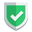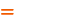我们相信：世界是美好的，你是我也是。平行空间的世界里面，不同版本的生活也在继续...

## 测试数据

``````import pandas as pd
df = pd.DataFrame([
('虎子', 5, "dog"),
('老许', 3, "bird"), ],
)
df2 = pd.DataFrame([
('虎子', 5, "dog"),
('老许', 3, "bird"),
('二赖子', 6, "fish"), ],
columns=("name", "age", "class")
)
df3 = pd.DataFrame([
('虎子', 5, "dog"),
('老许', 3, "bird"),
('二赖子', 6, "fish"), ],
index=["a1", "a2", "a3"],
columns=("name", "age", "class")
)``````

• 根据这个`dataframe`数据，对于`index``columns`是否进行了定义，可以选用`pandas.DataFrame.loc(index,columns)``pandas.DataFrame.iloc(index,columns)`这两个函数。
• `index``columns`默认是数字，但是如果被定义之后，就必须使用对应的字符串了，数字索引就对于`loc()`不生效，但是对于`iloc()`仍然生效。

## loc(index,columns)

`index,columns`，可以取值数字，也可以取值字母，这取决于`DataFrame`的索引情况。

``````# <class 'str'>
print(df.loc[0, 0])           # 虎子
# print(df2.loc[0,1])         # KeyError: 1
# print(df3.loc[0,1])         # KeyError: 1
print(df2.loc[0, "name"])     # 虎子
# print(df3.loc[0,"name"])    # KeyError: 0
print(df3.loc["a1", "name"])  # 虎子``````

``````# <class 'pandas.core.series.Series'>
a2 = df2.loc[:, "name"]
a3 = df2.loc[:2, "name"]
# a4 = df3.loc[1:2, "name"] # TypeError: cannot do slice indexing on Index with these indexers
a4 = df3.loc["a1":"a3", "name"]
a5 = df3.loc["a1":"a3", "name":"class"]``````

## iloc(index,columns)

``````# print(df2.iloc[0,"name"])  # ValueError: Can only index by location with a [integer, integer
print(df.iloc[0, 0])   # 虎子
print(df2.iloc[0, 1])  # 5
print(df3.iloc[0, 1])  # 5``````

``````# print(df2.iloc[1,"name"]) #虎子
print(df.iloc[:,1])
print(df2.iloc[2:,1])
print(df3.iloc[:2,1])
print(df3.iloc[:2,0:2])``````

## `.values`

``````# print(df.loc[1,2].values)     # AttributeError: 'str' object has no attribute 'values'
print(df.loc[:,2].values)       # ['dog' 'bird']
# print(df2.loc[0:2,2].values)  # KeyError: 2
print(df2.iloc[0:2,2].values)   # ['dog' 'bird']         <class 'numpy.ndarray'>
print(df2.iloc[0:2,0:2].values) # [['虎子' 5]['老许' 3]]  <class 'numpy.ndarray'>``````

## 修改数据

``df2.loc[df2.loc[:, 'name'] == "虎子", "ok"] = 1 ``

``````import pandas as pd
df = pd.DataFrame(
[
["虎子", "狗", 15],
["老许", "猫", 10],
],
columns=["姓名", "种类", "重量"],
)

# 修改行
df.iloc = ["小许", "猫", 3]
df.iloc[0, :] = ["二赖子", "狗", 16]
print(df)

# 修改列
df.iloc[:, 1] = ["dog", "cat"]
print(df)``````

``````    姓名  种类  重量
0  二赖子  狗   16
1   小许   猫   3

姓名   种类  重量
0  二赖子  dog   16
1   小许   cat   3``````

## 综述

loc根据情况，可数字，可字母，可范围根据情况，可数字，可字母，可范围
iloc必须是数字，或数字范围必须是数字，或数字范围

loc(index,column)字符串
loc(:,:)Series
loc(:,:).valuesndarray如果本文对您有帮助，或者节约了您的时间，欢迎打赏瓶饮料，建立下友谊关系。本博客不欢迎：各种镜像采集行为。请尊重原创文章内容，转载请保留作者链接。【福利】 腾讯云最新爆款活动！1核2G云服务器首年50元！【源码】本文代码片段及相关软件，请点此获取更多信息【绝密】秘籍文章入口，仅传授于有缘之人python    pandas